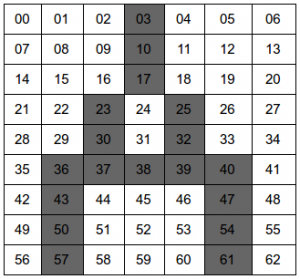# Artificial Intelligence – Self Organizing Map Simple/Best example with explanation

Self Organizing Map is a type of Artificial Neural Network that trains data using unsupervised learning. It can classify huge data based on the similarity of coding of data. It can be used to simply classify sample dataset such that whenever a new data is entered it provides where the data belongs to and can be added to that group. Being unsupervised learning, we don’t know the answer but we can know where it could group. Will need further assistance, what input is to be specific.
I had to present in this topic and found the perfect tutorial that gave me the best explanation.

After viewing the above tutorial, I wondered how this can be implemented into a real life example. So, I started searching for some already implemented code and how it would work. And found a python code that gave me how can anything be represented as binary and how we can make the relation. But at first we need how everything can be coded. From the python code I learned that the way anyone writes a character is a white space or black ink. Then I started visualizing every character as referred in the above link as table.So, what I guessed is that a character just either 0 or 1 and I can add it my samples. Same character can have multiple version of similar properties. So, each of the A’s there can be written as
Sample 1
001100000010000001000001010000101000111110010001001000101100011
Sample 2
000100000010000001000001010000101000111110010001001000100100010
Now, we have code for these A’s and we can have more samples of As and other alphabets. This is a simple example for character recognition, it doesn’t says that a new sample is A but the new A that almost looks like A will most probably group into the group which is on A. For example, for any other real life example say grouping flowers. We have to think of too many parameters such as petal length, width, color; sepal length, width, color. Now the problem here arises is how do we represent the data in such binary so that we can train data. Lets try to get few samples for any flower. This is a assumed sample to make you assume how to convert properties to binary format. It is not a huge science to convert but we need to think of the features that are important that can separates between flowers and SOM doesn’t say you what a new flower is, but it will point to the group of the flower that has most similar properties.
Lets assume 2 samples:

So, we have 2 samples. To represent these 2 as binary we need to change those number to decimal. If there is color then we can code the colors like red = 1, blue = 2, green = 3, purple = 4 and more. Now we have represented them all in decimal. Simplest way to represent them as decimal is by giving point(.) by some code and value behind point by another coding. Like 6.5 can be coded as simple mathematics 00110|0101. And further we can similarly code for all the values there. So the final coding for above sample 1 can be written as

This is just a coding and may not work because I haven’t analyzed which parameter has high priority but now we know how to change our data/image into binary coding. I have added a simple code on github for the sample I made for the class presentation. There on som.html, I have some sample I have kept here as below.

These are sample simple array of 0s and 1s for A, B, C and D. Similar other array for few more alphabets are added there. We know that we have 26 characters in capital case so we create a 2-dimensional array of 26 x 63. Where 26 are the classes and 63 is representation of a alphabet in binary which is 63 bit long.

• We have a new 2D array of 26 x 63 with some random weights
• We send each training set of data to each 2D array
• Euclidean distance (training set, individual class weight), where training set is 63 bit long and each class have 63 weights initialized
• Now we select the smallest one in the Euclidean distance and update the weight of the one with the smallest distance
• We do this process for another training set
• We repeat above step till we have good classification based on the type.

In my example on som.html we have a decay rate and it updates on each iteration. And we stop when the decay rate is low such that it does not have any more affect on further iterations. After the training, we find that the “A” is grouped to a class which is the position/index of our array. For my case, it grouped to class 19 as in screenshotNow let us create a new sample and test and try to find out which group it lies. It shouldn’t exact match but it will try to match that has the lowest Euclidean distance with the sample and group. And found the result asWe see that the one that matches lies in same group. It doesn’t say that it is 1, but it lies in that specific group. It will need further assistance in finding what is that group. You can ask me anything regarding this subject, I will try my best to reply as soon as possible.